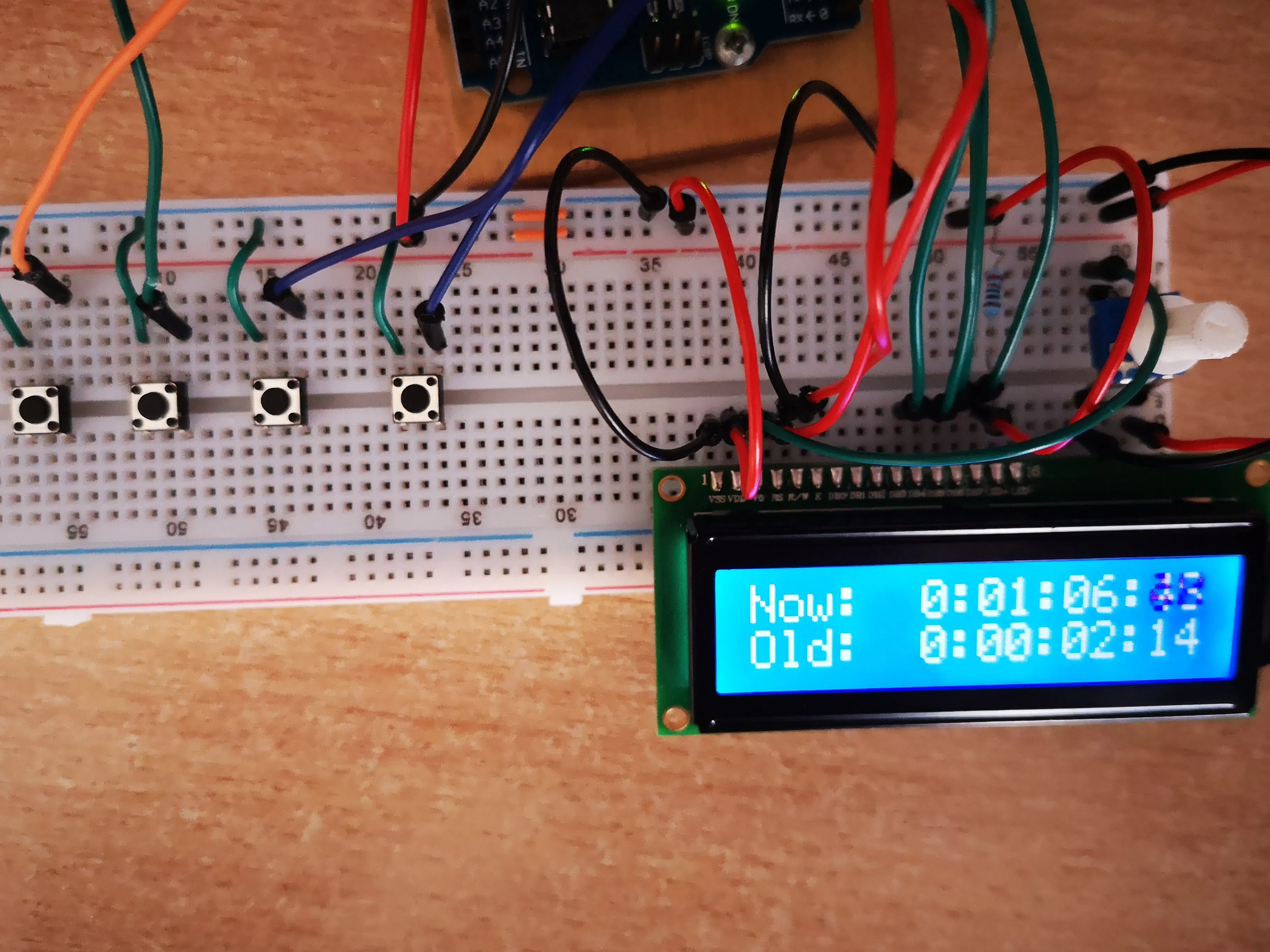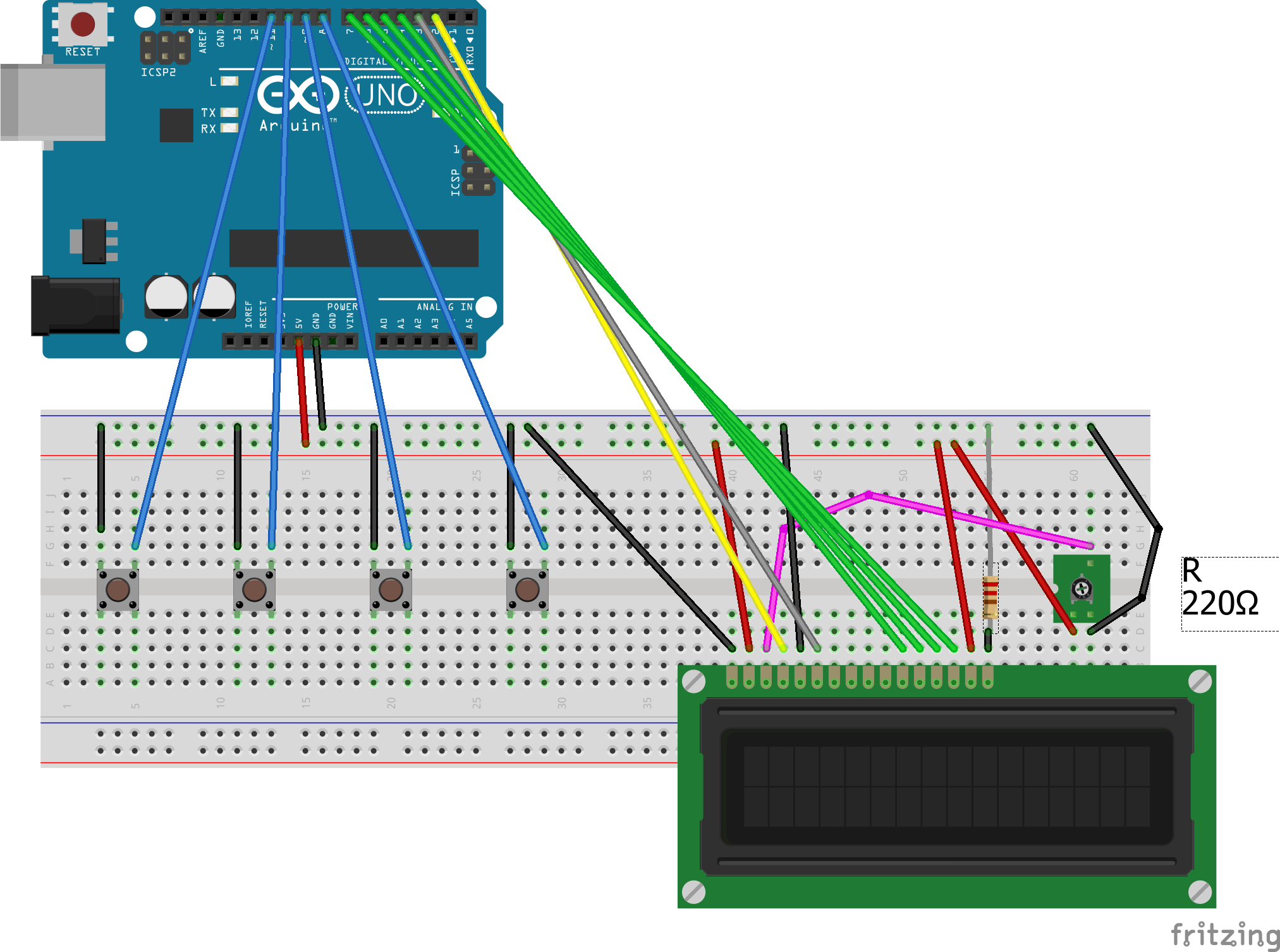# LCD Stopwatch, with Split time © GPL3+

A simple stopwatch that can store up to 4 split times.

• 7,753 views
• 13 respects

## Components and supplies

I wanted to create a simple stopwatch with a lcd, and wich could store 4 split time in memory.

How does it work:

This stopwatch has 4 buttons, in the schematic, from right to left: Start, Pause, Split (Partial time), and Scroll the split time.

Once you press the start button for the first time, the chronometer starts. If you press the pause button it will stop, very simple. If while it's running, you press the third button (Split), it will save that time in memory (in Old: ), and it will start a new split. When the clock is in pause mode, if you press the 4th button (scroll), you'll enter in the function to see the last 4 split time. Once you press start again, the chronometer just will start again. The code is explained in the comments, hope you willl enjoy :)

Tutorial for components used in the project:

- Potentiometer: https://arduinogetstarted.com/tutorials/arduino-potentiometer

## Code

##### CodeArduino
```#include <LiquidCrystal.h>
//PIN 5 R/W to Ground, for writing
//LCD Mode : 4 data pin
//Functions: Start, stop, saving up to 4 partial time in memory.
//10 millis = 1 hundredth of a second. The chronometer measures hour, minutes, seconds, and hundredths of a second.

//Buttons with internal Pullup, so if they get pressed we get LOW
const int start = 8;  //Start the stopwatch
const int pausa = 9;  //Pausa
const int partial = 10;  //Save a partial
const int scroll_partial = 11;  //If paused, check the 4 last saved partial
int x = 0; //Variable to manage the loop

//LCD
int lcdclear = 0; //this to variables are needed for managing
int Display = 0; //the display, to clear and print

//chronometer
int cents = 0;
int seconds = 0;
int minutes = 0;
int hours = 0;
const int interval = 10; //Every 10 milliseconds i increase  1 cent
unsigned long previousMillis = 0;
int c1, c2, s1, s2, m1, m2, h; //Variables used to put in the form
//h:m2m1:s2s1:c2c1

//Partial: I save 4 partial, that can be seen if stopwatch is stopped
int partial2; //penultimate partial (The one that stays in Old). The last partial stays in New:
int partial3;
int partial4;
int scrolling = 0; //Used to scroll the saved partial times

LiquidCrystal lcd(2, 3, 4, 5, 6, 7); // RS-Enable-D4-D5-D6-D7 in that digitalPin

void setup() {
pinMode(start, INPUT_PULLUP); //In the schematic from right to left
pinMode(pausa, INPUT_PULLUP);//there are start-pausa-partial-scroll_partial
pinMode(partial, INPUT_PULLUP);
pinMode(scroll_partial, INPUT_PULLUP);
lcd.begin(16,2);
lcd.print("Press start");

}

void loop() {
if (x == 0) {  //Initially is 0
while(digitalRead(start) == HIGH) {};  //Until i press the button, the chronometer doesn't start
x++; //When i press the button, i go out from this cycle, x++ and i cannot return here anymore
}
if (lcdclear == 0){   //Condition to clear the display, used in the various functions
lcd.clear();
lcdclear++;
}
if (Display == 0){ //Also this is used to clear display
lcd.home();
lcd.print("Now:  ");  //With this condition, i can print "Now: " one single time, otherwise the chronometer wouldn't be precise
Display++;
scrolling = 0; //When i exit from the partial menu, then if i go in the partial menu again i always get in PAR1 and PAR2
}
chronometer(); //At the end of every increase, i control if stop is pressed. And also if the partial function is pressed
pause();
f_partial();
}

void chronometer(void){   //This function print:   "New: Actual time"
unsigned long currentMillis = millis();  //If for the updating. If it is true, it means 1 cent of a second had passed. Update cents, minutes, seconds, hours and then i write on the lcd
if (currentMillis - previousMillis >= interval) {
previousMillis = currentMillis;
cents++;
if (cents == 100){
cents = 0;
seconds++;
if (seconds == 60) {
seconds = 0;
minutes++;
if (minutes == 60){
minutes = 0;
hours++;
if (hours == 24)
hours = 0;
}
}
}
int cent = cents;
int sec = seconds;
int minu = minutes; //Taking the digits separeted
h = hours;  //For the other funcionts, so i can put hours = 0 and h still is the last value
c1 = cent%10;
cent /= 10;
c2 = cent%10;
s1 = sec%10;
sec /= 10;
s2 = sec%10;
m1 = minu%10;
minu /= 10;
m2 = minu%10;
lcd.setCursor(6, 0);
lcd.print(h);
lcd.print(':');
lcd.print(m2);
lcd.print(m1);
lcd.print(':');
lcd.print(s2);
lcd.print(s1);
lcd.print(':');
lcd.print(c2);
lcd.print(c1);
}
}

void scrollPartial(void){
while(digitalRead(scroll_partial) == LOW) {}; //Debounce, as long as i press the button the real function doesn't start
if (scrolling == 0) { //Visualize the last 2 partials
lcd.clear();
lcd.home();
lcd.print("PAR1:");
lcd.setCursor(6, 0);
lcd.print(h);
lcd.print(':');
lcd.print(m2);
lcd.print(m1);
lcd.print(':');
lcd.print(s2);
lcd.print(s1);
lcd.print(':');
lcd.print(c2);
lcd.print(c1);
lcd.setCursor(0, 1);
lcd.print("PAR2:");
lcd.setCursor(6, 1);
lcd.print(partial2);
lcd.print(':');
lcd.print(partial2);
lcd.print(partial2);
lcd.print(':');
lcd.print(partial2);
lcd.print(partial2);
lcd.print(':');
lcd.print(partial2);
lcd.print(partial2);
Display = 0; //When i press start the display must be cleared
lcdclear = 0; //When i press start the display must be cleared
cents = seconds = minutes = hours  = 0;
scrolling++;
}
else if (scrolling == 1){ //Visualize 3th and 4th partial
lcd.clear();
lcd.home();
lcd.print("PAR3:");
lcd.setCursor(6, 0);
lcd.print(partial3);
lcd.print(':');
lcd.print(partial3);
lcd.print(partial3);
lcd.print(':');
lcd.print(partial3);
lcd.print(partial3);
lcd.print(':');
lcd.print(partial3);
lcd.print(partial3);
lcd.setCursor(0, 1);
lcd.print("PAR4:");
lcd.setCursor(6, 1);
lcd.print(partial4);
lcd.print(':');
lcd.print(partial4);
lcd.print(partial4);
lcd.print(':');
lcd.print(partial4);
lcd.print(partial4);
lcd.print(':');
lcd.print(partial4);
lcd.print(partial4);
Display = 0; //When i press start the display must be cleared
lcdclear = 0; //When i press start the display must be cleared
cents = seconds = minutes = hours  = 0;
scrolling = 0;

}

}

void pause(void){  //If pause is pressed, i stop in this function until start doesn't get pressed again
return;
else if (digitalRead(pausa) == LOW){ //Stuck in this cycle until i press start
if (digitalRead(scroll_partial) == LOW) //If i press the button for seeing the partial, i enter in that function
scrollPartial();    //When scrollPartial() ends, i'm still in this function, so if i press start the chronometer starts back normal
}
}
}

void f_partial(void){   //If this button is pressed, i put the current value of New in Old, and a new crhonometer starts
return;
else if (digitalRead(partial) == LOW ){
lcd.clear();
lcd.setCursor(0, 1); //The values calculated in the function chronometer can be used,  h,m,s,c
lcd.print("Old: ");
lcd.setCursor(6, 1);
lcd.print(h);
lcd.print(':');
lcd.print(m2);
lcd.print(m1);
lcd.print(':');
lcd.print(s2);
lcd.print(s1);
lcd.print(':');
lcd.print(c2);
lcd.print(c1);   //When i come here, i've got the old values for h,m,s,c,                       i save it in the partial array
Display = 0;   //The new is written again
cents = 0;
seconds = 0;
minutes = 0;
hours = 0;

partial4 = partial3; //Partial4[] is updated with the old partial3[]
partial4 = partial3;
partial4 = partial3;
partial4 = partial3;
partial4 = partial3;
partial4 = partial3;
partial4 = partial3;

partial3 = partial2; //Partial3[] is updated with the old partial2[]
partial3 = partial2;
partial3 = partial2;
partial3 = partial2;
partial3 = partial2;
partial3 = partial2;
partial3 = partial2;

partial2 = h;    //Update partial2 with OLD
partial2 = m2;
partial2 = m1;
partial2 = s2;
partial2 = s1;
partial2 = c2;
partial2 = c1;

while(digitalRead(partial) == LOW) {}; //Debounce, until i press the button i stay here
}
}
```

## Schematics#### Published on

December 26, 2019

#### Members who respect this project

See similar projects
you might like

#### Arduino Nano LCD Stopwatch Without Potentiometer

Project tutorial by TechWithZan

• 23,790 views
• 26 respects

#### LCD with DS3231 Real Time Clock Module

Project showcase by Tan See Youu

• 62,881 views
• 48 respects

#### Stopwatch and Lap Timer with Arduino

Project showcase by LAGSILVA

• 32,879 views
• 61 respects

#### Stopwatch with Arduino starter kit

Project showcase by mingmike

• 10,306 views
• 12 respects

#### Clock Set Date Time

Project tutorial by Tittiamo

• 105,477 views